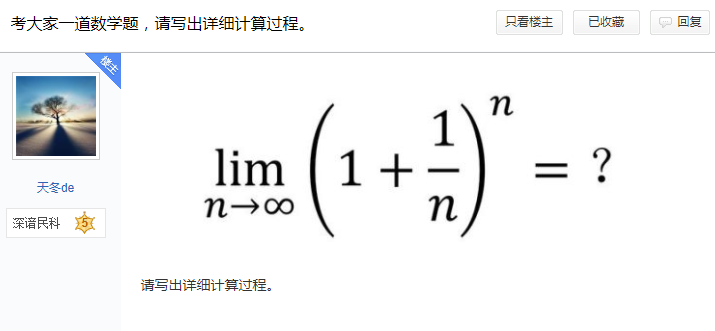5G时代下一个聚合的编程学习网

# 走一走 欧拉先生 走过 的 路dy / dx = y     的 意义 是   y ′= y ，   也就是 导数 和 原函数 一样，  是 同一个 函数，   也就是 存在一个   y = f ( x )  ，  使得   f ‘ ( x ) = f ( x )   。

[ e ^ ( x + ⊿ x ) – e ^ x ]  / ⊿ x    =    e ^ x     ,     ⊿ x -> 0

e ^ ( x + ⊿ x ) – e ^ x    =    e ^ x   *   ⊿ x

e ^ x   *   e ^ ⊿ x    –    e ^ x     =     e ^ x   *   ⊿ x

e ^ ⊿ x   –   1    =    ⊿ x

e ^ ⊿ x     =     1   +   ⊿ x

e   =    ( 1  +  ⊿ x )  ^  ( 1 / ⊿ x )

e   =   ( 1  +  1 / n )  ^ n    ,      n -> 无穷